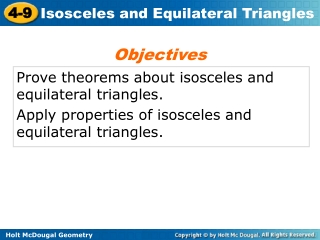DownloadDownload PresentationProve theorems about isosceles and equilateral triangles.

# Prove theorems about isosceles and equilateral triangles.

Télécharger la présentation## Prove theorems about isosceles and equilateral triangles.

- - - - - - - - - - - - - - - - - - - - - - - - - - - E N D - - - - - - - - - - - - - - - - - - - - - - - - - - -
##### Presentation Transcript

1. Objectives Prove theorems about isosceles and equilateral triangles. Apply properties of isosceles and equilateral triangles.

2. Vocabulary legs of an isosceles triangle vertex angle base base angles

3. Recall that an isosceles triangle has at least two congruent sides. _____ - The congruent sides of an isosceles triangle. ____________– The angle formed by the legs. ______ – The side opposite the vertex angle. ______________– The two angles that have the base as a side.  is the vertex angle.  and  are the base angles.

4. Reading Math The Isosceles Triangle Theorem is sometimes stated as “Base angles of an isosceles triangle are congruent.”

5. The length of YX is 20 feet. Explain why the length of YZ is the same. Example 1A: Astronomy Application

6. Example 3A: Using Properties of Equilateral Triangles Find the value of x.

7. Example 3B: Using Properties of Equilateral Triangles Find the value of y.